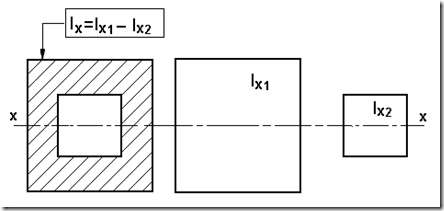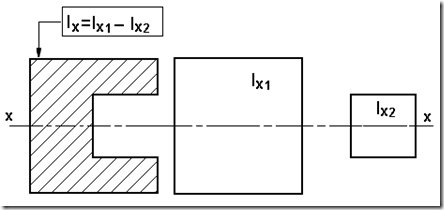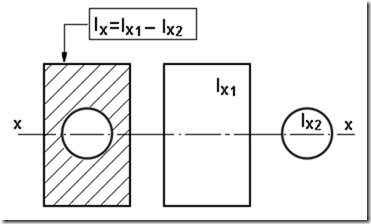" /> Tiled Sectional Moment of Inertia | TN Mesin
Home > English > Tiled Sectional Moment of Inertia

# Tiled Sectional Moment of Inertia

If the cross-section of a field made up of a cross-sectional arranged symmetrically to the axis line, then the moment of inertia is a number or a reduction of the cross section composed penapang. For example, two squares forming a cross-hatched as shown in the picture, it is the moment of inertia Ix = Ix1 – Ix2. Stackable cross-section of two squares with the position symmetrical to the axis xx.Stackable cross-section of two squares with the position symmetrical to the axis xx with squares second position located on the side.Stackable cross-section of the two forms of the rectangular area with the circle, the position of these two areas symmetrical to the axis xx.Composite cross-section of the three forms of the rectangular area with the position of these three areas symmetrical to the axis xx.Figure 5.21 The moment of inertia of a cross-decker